# 量子漫步搜索算法

## 1. 经典马尔可夫链

P= \begin{pmatrix} 0.1 & 0.3 & 0.3\\ 0.1 & 0.1 & 0.2 \\ 0.8 & 0.6 & 0.5 \end{pmatrix} \label{eq:matrix_example}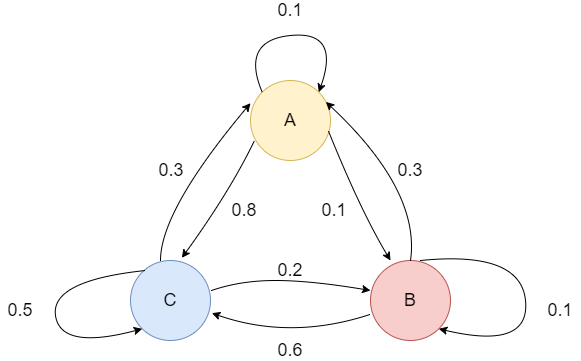## 2. 量子漫步

### 2.A 硬币态量子漫步

H = \frac{1}{\sqrt{2}} \begin{bmatrix} 1 & 1 \\ 1 & -1 \end{bmatrix}

Grover硬币是Grover算法中的Grover扩散算子。我们把它定义为

G = \begin{bmatrix} \frac{2}{n} -1 & \frac{2}{n} & \ldots & \frac{2}{n}\\ \frac{2}{n} & \frac{2}{n} - 1 & \ldots & \frac{2}{n} \\ \vdots & \vdots & \ddots & \vdots \\ \frac{2}{n} & \frac{2}{n} & \ldots & \frac{2}{n} -1 \end{bmatrix}S \ket{0}\ket{j} = \ket{0}\ket{j+1}
S \ket{1}\ket{j} = \ket{1}\ket{j-1}

U = SC,

\ket{\psi (t)} = U^t \ket{\psi(0)},

### 2.B Szegedy量子漫步

\ket{\psi_j} := \sum_{k=1}^N \sqrt{P_{kj}} \ket{j,k}, \; j=1,...,N

\Pi := \sum_{j=1}^N \ket{\psi_j} \bra{\psi_j} \label{eq:sz_pi}

S := \sum_{j,k=1}^N \ket{j,k} \bra{k,j} \label{eq:sz_s}

U := S(2 \Pi - 1), \label{eq:sz_op}

## 3.示例:在超立方体上实现量子漫步

\big\{ \ket{a,\vec{v}}, 0 \leq a \leq n-1, \vec{v} \in \{(00...00), (00...01), ....., (11...11 )\} \big\}.

S \ket{a} \ket{\vec{v}} = \ket{a} \ket{\vec{v} \oplus \vec{e}_a}.

U = SG.

# Importing standard Qiskit libraries
from qiskit import QuantumCircuit, execute, Aer, IBMQ, QuantumRegister, ClassicalRegister
from qiskit.compiler import transpile, assemble
from qiskit.tools.jupyter import *
from qiskit.visualization import *
from qiskit.circuit.library import QFT
from numpy import pi
from qiskit.quantum_info import Statevector
from matplotlib import pyplot as plt
import numpy as np


one_step_circuit = QuantumCircuit(6, name=' ONE STEP')
# Coin operator
one_step_circuit.h([4,5])
one_step_circuit.z([4,5])
one_step_circuit.cz(4,5)
one_step_circuit.h([4,5])
one_step_circuit.draw()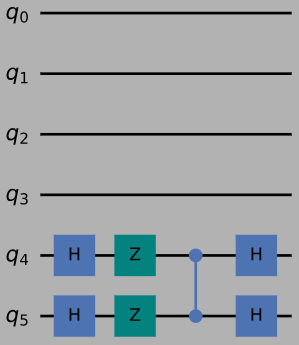# Shift operator function for 4d-hypercube
def shift_operator(circuit):
for i in range(0,4):
circuit.x(4)
if i%2==0:
circuit.x(5)
circuit.ccx(4,5,i)

shift_operator(one_step_circuit)

one_step_gate = one_step_circuit.to_instruction()
one_step_circuit.draw()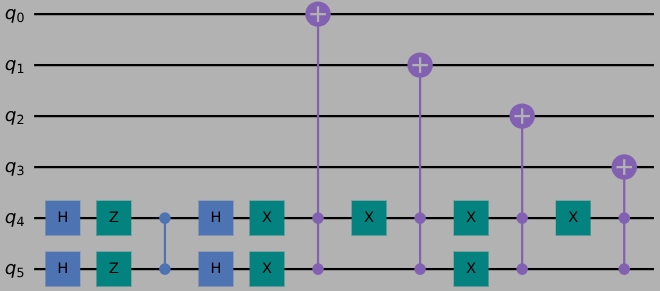## 4. 量子漫步搜索算法

\ket{G} = \frac{1}{\sqrt{|M|}} \sum_{x \in M} \ket{x} \ket{p_x}, \; \ket{B} = \frac{1}{\sqrt{N-|M|}} \sum_{x \notin M} \ket{x} \ket{p_x},

1. 设置初始状态 \ket{U} = \frac{1}{\sqrt{N}} \sum_{x} \ket{x} \ket{p_x} = \sin{\theta} \ket{G} + \cos{\theta} \ket{B} ，所有边的均匀叠加

2. 重复执行 O(1/\sqrt{\epsilon}) 次:

(a) 通过 \ket{B} 进行反射

(b) 通过 \ket{U} 进行反射

3. 在计算的基础上做测量

\begin{align} \label{eq:mapping_1} \ket{U} &\mapsto \ket{U}, \: \text{和} \\ \ket{\psi} &\mapsto -\ket{\psi}, \: \forall \ket{\psi} \text{的特征向量张成的空间 $W(P)$ 正交于 $\ket{U}$ } \label{eq:mapping_2} \end{align}

1 ，即 \ket{U} 。这由 \theta_1=0 给出， R(P) 将通过添加一个带有辅助量子比特的寄存器来找到这个向量 \ket{U} ，并以 O(1/\sqrt{\delta}) 的精度执行相位估计，其中 δ P 的谱隙。为此，我们需要应用 W(P) O(1/\sqrt{\delta}) 次。设 \ket{w} w (P) 的特征向量，特征值 e^{\pm 2i\theta_j} 。假设 \tilde{\theta_j} 是由相位估计给出的 \tilde{\theta_j} 的最佳近似。操作 R(P)

\ket{w} \ket{0} \mapsto \ket{w} \ket{\tilde{\theta_j}} \mapsto (-1)^{|\tilde{\theta_j} \neq 0|} \ket{w} \ket{\tilde{\theta_j}} \mapsto (-1)^{|\tilde{\theta_j} \neq 0|} \ket{w} \ket{0}

## 5. 示例:4维超立方体上的量子漫步搜索

one_step_circuit.inverse().draw()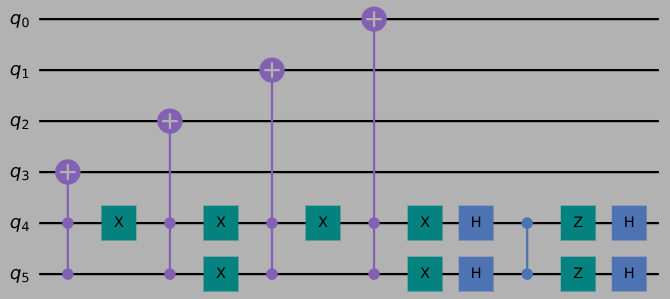# Make controlled gates
inv_cont_one_step = one_step_circuit.inverse().control()
inv_cont_one_step_gate = inv_cont_one_step.to_instruction()
cont_one_step = one_step_circuit.control()
cont_one_step_gate = cont_one_step.to_instruction()


inv_qft_gate = QFT(4, inverse=True).to_instruction()
qft_gate = QFT(4, inverse=False).to_instruction()

QFT(4, inverse=True).decompose().draw("mpl")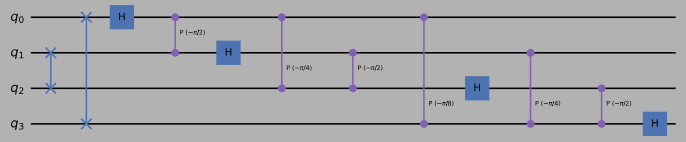phase_circuit =  QuantumCircuit(6, name=' phase oracle ')
# Mark 1011
phase_circuit.x(2)
phase_circuit.h(3)
phase_circuit.mct([0,1,2], 3)
phase_circuit.h(3)
phase_circuit.x(2)
# Mark 1111
phase_circuit.h(3)
phase_circuit.mct([0,1,2],3)
phase_circuit.h(3)
phase_oracle_gate = phase_circuit.to_instruction()
# Phase oracle circuit
phase_oracle_circuit =  QuantumCircuit(11, name=' PHASE ORACLE CIRCUIT ')
phase_oracle_circuit.append(phase_oracle_gate, [4,5,6,7,8,9])
phase_circuit.draw()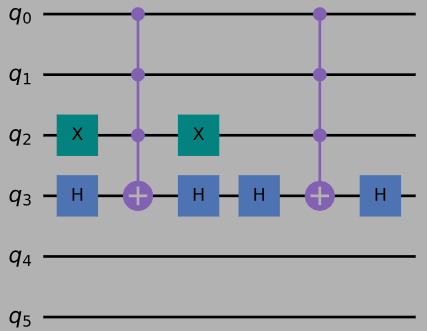# Mark q_4 if the other qubits are non-zero
mark_auxiliary_circuit = QuantumCircuit(5, name=' mark auxiliary ')
mark_auxiliary_circuit.x([0,1,2,3,4])
mark_auxiliary_circuit.mct([0,1,2,3], 4)
mark_auxiliary_circuit.z(4)
mark_auxiliary_circuit.mct([0,1,2,3], 4)
mark_auxiliary_circuit.x([0,1,2,3,4])

mark_auxiliary_gate = mark_auxiliary_circuit.to_instruction()
mark_auxiliary_circuit.draw()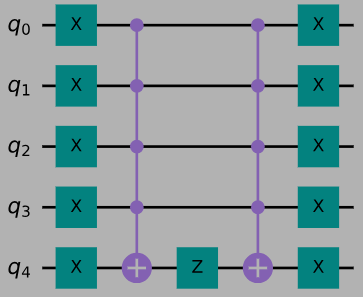# Phase estimation
phase_estimation_circuit = QuantumCircuit(11, name=' phase estimation ')
phase_estimation_circuit.h([0,1,2,3])
for i in range(0,4):
stop = 2**i
for j in range(0,stop):
phase_estimation_circuit.append(cont_one_step, [i,4,5,6,7,8,9])

# Inverse fourier transform
phase_estimation_circuit.append(inv_qft_gate, [0,1,2,3])

# Mark all angles theta that are not 0 with an auxiliary qubit
phase_estimation_circuit.append(mark_auxiliary_gate, [0,1,2,3,10])

# Reverse phase estimation
phase_estimation_circuit.append(qft_gate, [0,1,2,3])

for i in range(3,-1,-1):
stop = 2**i
for j in range(0,stop):
phase_estimation_circuit.append(inv_cont_one_step, [i,4,5,6,7,8,9])
phase_estimation_circuit.barrier(range(0,10))
phase_estimation_circuit.h([0,1,2,3])

# Make phase estimation gate
phase_estimation_gate = phase_estimation_circuit.to_instruction()
phase_estimation_circuit.draw()


# Implementation of the full quantum walk search algorithm
theta_q = QuantumRegister(4, 'theta')
node_q = QuantumRegister(4, 'node')
coin_q = QuantumRegister(2, 'coin')
auxiliary_q = QuantumRegister(1, 'auxiliary')
creg_c2 = ClassicalRegister(4, 'c')
circuit = QuantumCircuit(theta_q, node_q, coin_q, auxiliary_q, creg_c2)
# Apply Hadamard gates to the qubits that represent the nodes and the coin
circuit.h([4,5,6,7,8,9])
iterations = 2

for i in range(0,iterations):
circuit.append(phase_oracle_gate, [4,5,6,7,8,9])
circuit.append(phase_estimation_gate, [0,1,2,3,4,5,6,7,8,9,10])

circuit.measure(node_q, creg_c2)
circuit.measure(node_q, creg_c2)
circuit.measure(node_q, creg_c2)
circuit.measure(node_q, creg_c2)
circuit.draw()


backend = Aer.get_backend('qasm_simulator')
job = execute( circuit, backend, shots=1024 )
hist = job.result().get_counts()
plot_histogram( hist )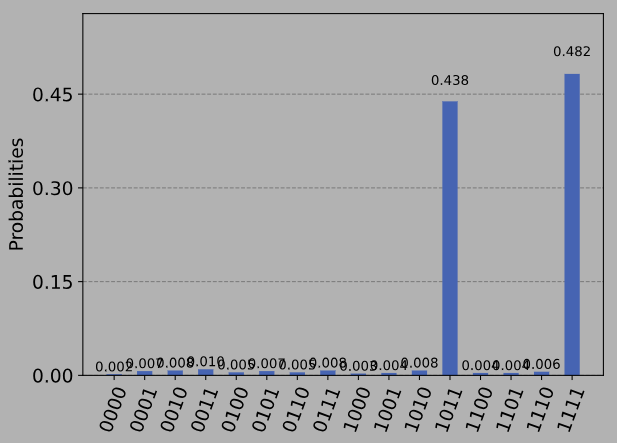## 6. 参考文献

1. Renato Portugal. Quantum Walks and Search Algorithms. New York, NY: Springer New York, 2013
2. Markus G. Kuhn.Some Introductory Notes on Quantum Computing. Apr. 2000
3. Thomas G. Wong. “Equivalence of Szegedy’s and coined quantum walks”. In: Quantum InformationProcessing 16.9 (July 2017). ISSN: 1573-1332. DOI:10.1007/s11128-017-1667-y. URL:http://dx.doi.org/10.1007/s11128-017-1667-y.37
4. Ronald de Wolf. Quantum Computing: Lecture Notes. 2021. arXiv:1907.09415 [quant-ph]
import qiskit.tools.jupyter
%qiskit_version_table


### 版本信息

Qiskit Software Version
qiskit-terra 0.18.0
qiskit-aer 0.8.2
qiskit-ignis 0.6.0
qiskit-ibmq-provider 0.15.0
qiskit-aqua 0.9.4
qiskit 0.28.0
System information
Python 3.7.7 (default, May 6 2020, 04:59:01) [Clang 4.0.1 (tags/RELEASE_401/final)]
OS Darwin
CPUs 8
Memory (Gb) 32.0

Tue Oct 05 13:51:31 2021 BST